Ore_algebra, a Package for Skew Operators

by Frederic Chyzak, Algorithms Project, INRIA, France

Frederic.Chyzak@inria.fr

Several algorithms for integration and summation have a natural description in terms of linear differential and difference operators, which in turn are well described by skew (or Ore) polynomials. This was the starting point for the Ore_algebra package.

> with(Ore_algebra);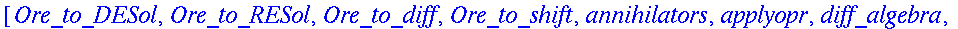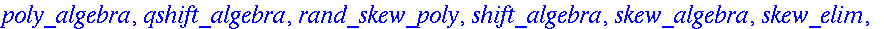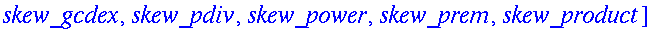To work with an Ore algebra, we first have to declare it. The package creates a table which implements and remembers the
operations in this algebra. Here is the example of the algebra of linear differential operators in the differential operator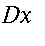with (rational) coefficients in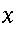.

> A:=diff_algebra([Dx,x]);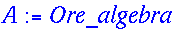(This is the name of the table.)

Although the usual product in Maple is commutative, we use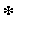to denote skew products with the convention that powers ofare on the right.

Multiplication of operators is obtained by the function skew_product.

> P1:=x*Dx^2-1: P2:=Dx-x: P1_P2:=skew_product(P1,P2,A);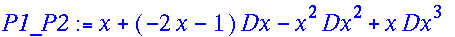Remember that in skew algebras of linear operators, factorizations are seldom unique.

> DEtools[DFactor](P1_P2,[Dx,x]);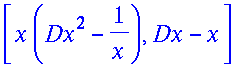(Univariate) Ore polynomial rings have a Euclidean algorithm. This is implemented in the following routines: skew_pdiv , skew_prem , skew_gcdex and skew_elim . An extension to the multivariate case is available.

As a first application, we show how the package can be used to derive a proof of the Reed-Dawson identity:

> U[n]=Sum(binomial(n,k)*binomial(2*k,k)*(-2)^(n-k),k=0..n);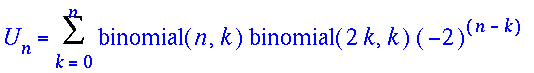is

> binomial(n,n/2);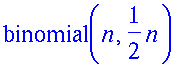for even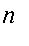and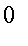for odd.

Let us introduce an algebra of linear recurrence operators inand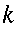.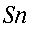and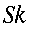denote the shift operators inandrespectively.

> A:=shift_algebra([Sn,n],[Sk,k]):

We compute first order recurrences satisfied by the summand (which we denote by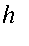).

> h:=binomial(n,k)*binomial(2*k,k)*(-2)^(n-k);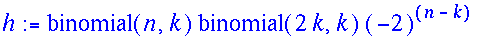> Sn-normal(applyopr(Sn,h,A)/h,expanded);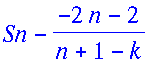> Sk-normal(applyopr(Sk,h,A)/h,expanded);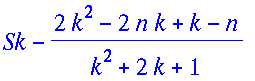> G:=map(numer,{%%,%}):

We eliminatebetween these operators by computing their greatest common right divisor.

> GCD:=skew_gcdex(op(G),k,A);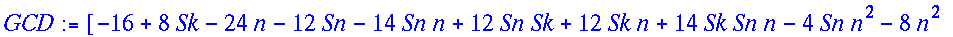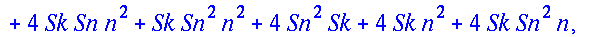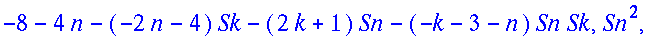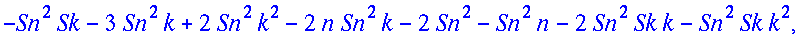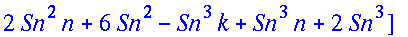> series(GCD,Sk=1);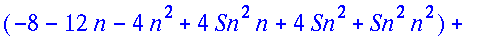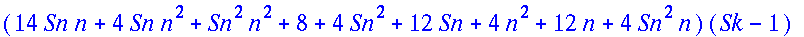The factorcorresponds to taking a finite difference. Definite summation forover all integers yields a telescoping series which collapses to 0. We thus get:

> Sum(applyopr(coeff(%,Sk-1,0),u[n,k],A),k=-infinity..infinity)=0;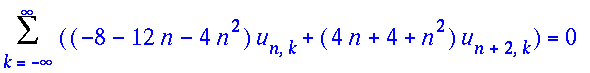Therefore, the sum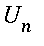satisfies

> collect(primpart(coeff(%%,Sk-1,0),Sn),Sn,normal);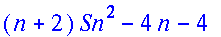> applyopr(%,U[n],A);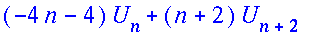Now, the announced result follows from initial conditions:

For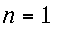,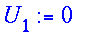so that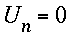for odd.

For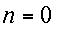,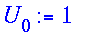so that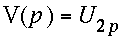is given by

> 2*(p+1)*V(p+1)-4*(2*p+1)*V(p);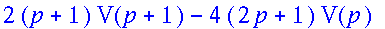> LREtools[hypergeomsols](%,V(p),{V(0)=1},output=basis);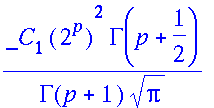which is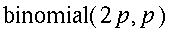.

As a more advanced application, we derive contiguity relations for Gauss hypergeometric function. This function is known to Maple as:

> f:=hypergeom([a,b],[c],z);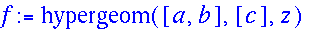It is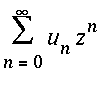for

> u:=pochhammer(a,n)*pochhammer(b,n)/pochhammer(c,n)/n!:

When considering the summand, we introduce the following algebra.

> A:=shift_algebra([Sn,n],[Sa,a],comm={b,c}):

The bivariate sequence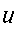vanishes at both following operators:

> G:={(c+n)*(n+1)*Sn-(a+n)*(b+n),a*Sa-(a+n)}:

> normal(map(applyopr,G,u,A),expanded);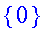(There are general algorithms to find such operators.)

From the previous first order recurrences, we derive relations on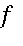in the mixed differential difference algebra

> A:=skew_algebra(diff=[Dz,z],shift=[Sa,a],comm={b,c}):

The equations are obtained by multiplication of the recurrences by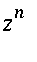, followed by summation over all non-negative. Formally, this corresponds to changinginto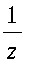andinto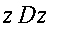.

> map(proc(p)
skew_product(skew_power(z*Dz,j,A),1/z^i,A),
j=0..degree(coeff(p,Sn,i),n)),
i=0..degree(p,Sn)),Dz,factor)
end,G);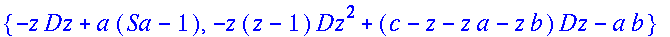Therefore, we set:

> P:=z*(1-z)*Dz^2+(c-(a+b+1)*z)*Dz-a*b:

> H:=z*Dz/a+1:

> G:={P,numer(Sa-H)};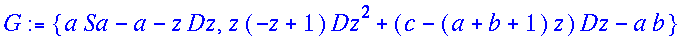The linear differential operator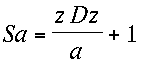is called a step-up operator. It relates the forward shift ofto derivatives of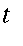by the following equation

> hypergeom([a+1,b],[c],z)=z/a*diff(hypergeom([a,b],[c],z),z)+hypergeom([a,b],[c],z);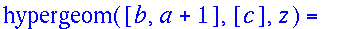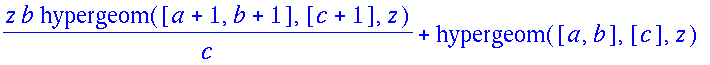The elimination of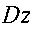between this step-up operator and the differential equation yields a contiguity relation for, i.e., a purely recurrence equation. It is obtained by the extended skew gcd algorithm:

> C:=collect(skew_elim(P,numer(Sa-H),Dz,A),Sa,factor);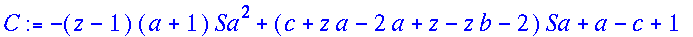In other words, Gauss hypergeometric function satisfies the following equation:

> applyopr(C,hypergeom([a,b],[c],z),A)=0;

More interestingly, the extended Euclidean algorithm yields a step-down operator for, i.e., a relation between an inverse shift ofand its derivatives. This is obtained by computing an inverse of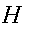modulo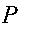.

> GCD:=skew_gcdex(P,numer(H),Dz,A);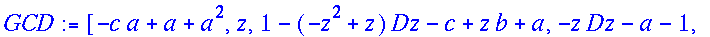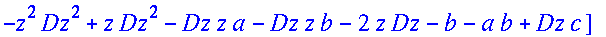From this result, we have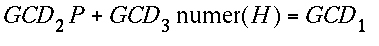and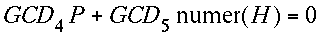. In particular,

> B:=collect((a-1)*subs(a=a-1,GCD/GCD),Dz,factor);

is the step-down operator:

> hypergeom([a-1,b],[c],z)=z*(1-z)*diff(hypergeom([a,b],[c],z),z)/(a-c)+(c-a-z*b)*hypergeom([a,b],[bc],z)/(c-a);

There is also an algorithm to compute (one-sided) Groebner bases in Ore algebras. This will be demonstrated in the presentation of the Groebner package.